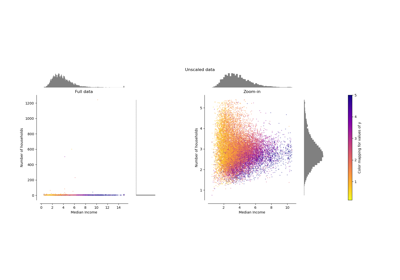# sklearn.preprocessing.minmax_scale¶

sklearn.preprocessing.minmax_scale(X, feature_range=(0, 1), *, axis=0, copy=True)[source]

Transform features by scaling each feature to a given range.

This estimator scales and translates each feature individually such that it is in the given range on the training set, i.e. between zero and one.

The transformation is given by (when axis=0):

X_std = (X - X.min(axis=0)) / (X.max(axis=0) - X.min(axis=0))
X_scaled = X_std * (max - min) + min


where min, max = feature_range.

The transformation is calculated as (when axis=0):

X_scaled = scale * X + min - X.min(axis=0) * scale
where scale = (max - min) / (X.max(axis=0) - X.min(axis=0))


This transformation is often used as an alternative to zero mean, unit variance scaling.

Read more in the User Guide.

New in version 0.17: minmax_scale function interface to sklearn.preprocessing.MinMaxScaler.

Parameters
Xarray-like of shape (n_samples, n_features)

The data.

feature_rangetuple (min, max), default=(0, 1)

Desired range of transformed data.

axisint, default=0

Axis used to scale along. If 0, independently scale each feature, otherwise (if 1) scale each sample.

copybool, default=True

Set to False to perform inplace scaling and avoid a copy (if the input is already a numpy array).

MinMaxScaler
Performs scaling to a given range using theTransformer API (e.g. as part of a preprocessing sklearn.pipeline.Pipeline).
## Examples using sklearn.preprocessing.minmax_scale¶